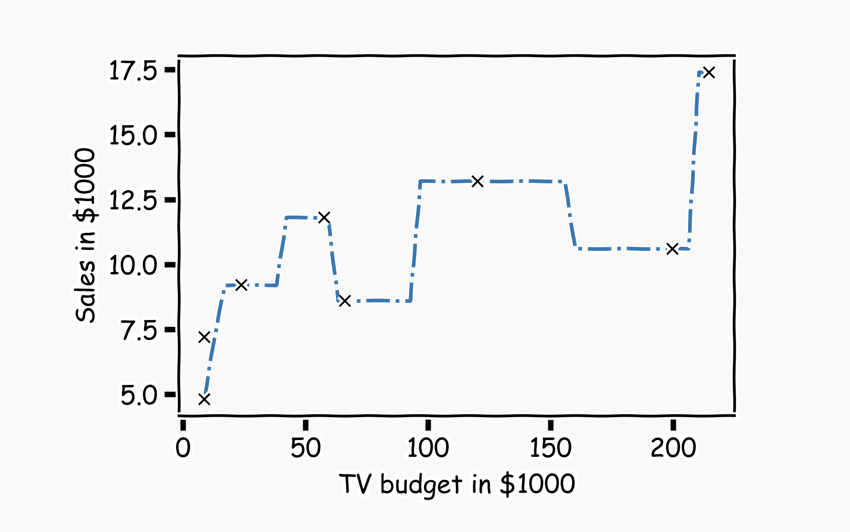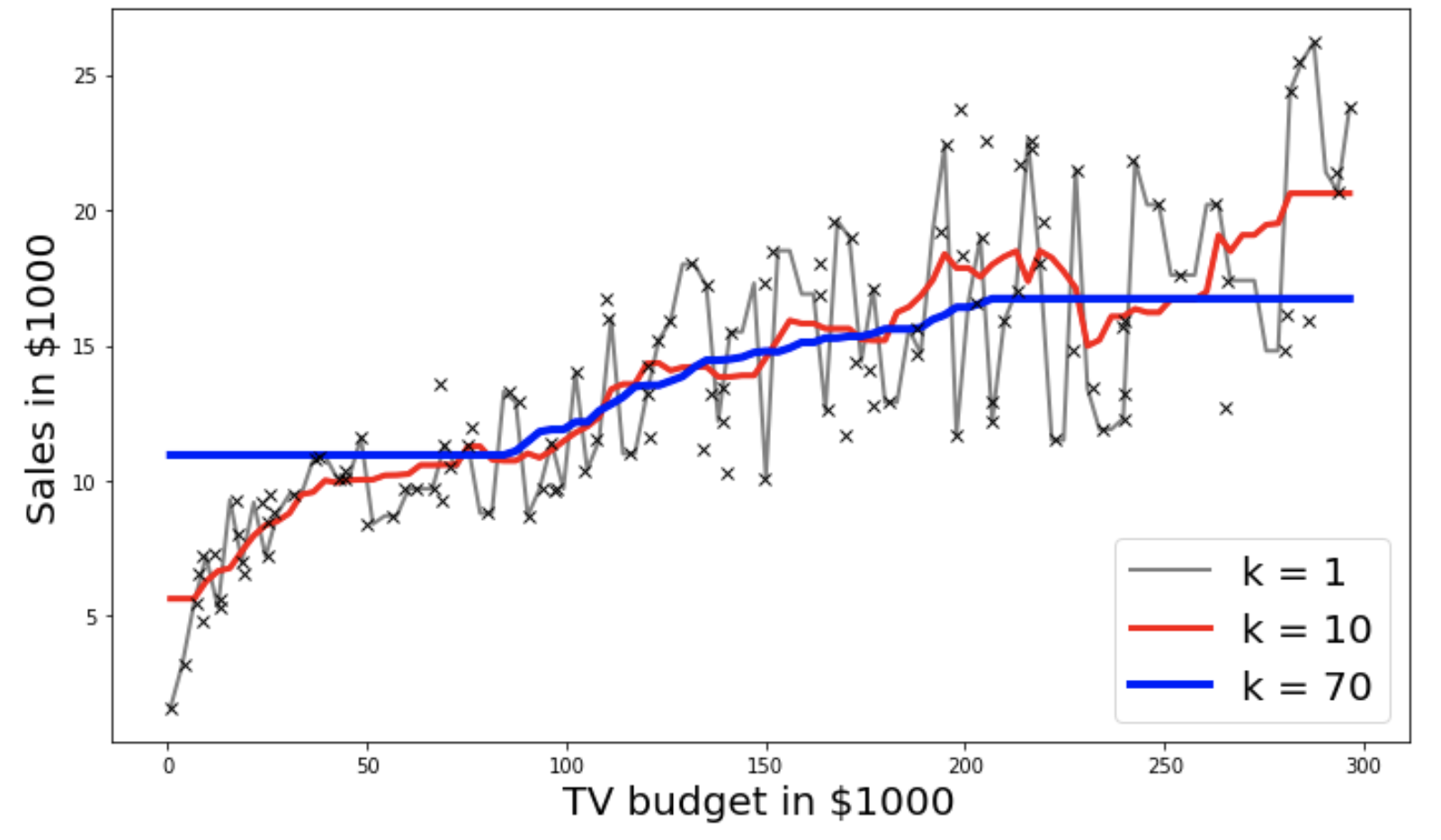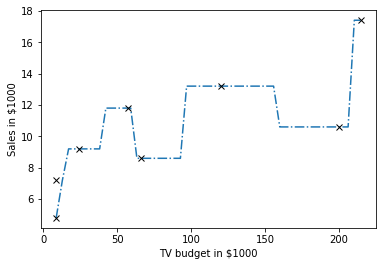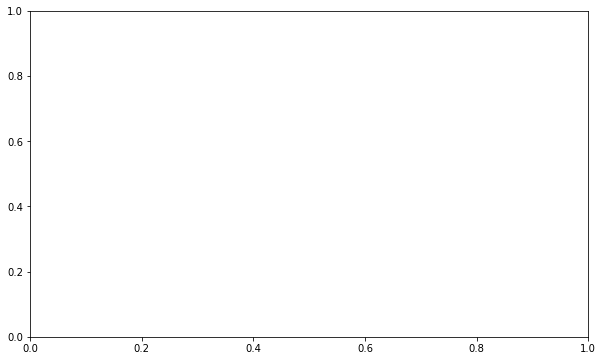# Title¶

Exercise: A.2 - Simple kNN regression

# Description¶

The goal of this exercise is to re-create the plots below from the lecture.In :
# Instructions:
Part 1 **KNN by hand for k=1**

- Get a subset of the data from row 5 to row 13
- Apply the kNN algorithm by hand and plot the first graph as given above.

Part 2 **Using sklearn package**
- Split the data into train and test sets using train_test_split() function
- Select k_list as possible k values ranging from 1 to 70.
- For each value of k in k_list:
- Use sklearn KNearestNeighbors() to fit train data
- Predict on the test data
- Use the helper code to get the second plot above for k=1,10,70

# Hints:
<a href="https://numpy.org/doc/stable/reference/generated/numpy.argsort.html" target="_blank">np.argsort()a> : Returns the indices that would sort an array.

<a href="https://pandas.pydata.org/pandas-docs/stable/reference/api/pandas.DataFrame.iloc.html" target="_blank">df.iloc[]a> : Returns a subset of the dataframe that is contained in the column range passed as the argument

<a href="https://pandas.pydata.org/pandas-docs/stable/reference/api/pandas.DataFrame.values.html" target="_blank">df.valuesa> : Returns a Numpy representation of the DataFrame.

<a href="https://pandas.pydata.org/pandas-docs/stable/reference/api/pandas.DataFrame.idxmin.html" target="_blank">pd.idxmin()a> : Returns index of the first occurrence of minimum over requested axis.

<a href="http://pageperso.lif.univ-mrs.fr/~francois.denis/IAAM1/numpy-html-1.14.0/reference/generated/numpy.ndarray.min.html" target="_blank">np.min()a> : Returns the minimum along a given axis.

<a href="https://numpy.org/doc/stable/reference/generated/numpy.ndarray.max.html" target="_blank">np.max()a> : Returns the maximum along a given axis.

<a href="https://numpy.org/devdocs/reference/generated/numpy.zeros.html" target="_blank">np.zeros()a> : Returns a new array of given shape and type, filled with zeros.

<a href="http://scikit-learn.org/stable/modules/generated/sklearn.model_selection.train_test_split.html" target="_blank">train_test_split(X,y)a> : Split arrays or matrices into random train and test subsets.

<a href="https://numpy.org/doc/stable/reference/generated/numpy.linspace.html" target="_blank">np.linspace()a> : Returns evenly spaced numbers over a specified interval.

<a href="https://scikit-learn.org/stable/modules/generated/sklearn.neighbors.KNeighborsRegressor.html" target="_blank">KNeighborsRegressor(n_neighbors=k_value)a> : Regression-based on k-nearest neighbors.

Note: This exercise is **auto-graded and you can try multiple attempts.**

In :
# take a quick look of the dataset


Out:
0 230.1 37.8 69.2 22.1
1 44.5 39.3 45.1 10.4
2 17.2 45.9 69.3 9.3
3 151.5 41.3 58.5 18.5
4 180.8 10.8 58.4 12.9
5 8.7 48.9 75.0 7.2

## Part 1: KNN by hand for $k=1$¶

In :
# Get a subset of the data rows 6 to 13 and only TV advertisement.
# The first row in the dataframe is the first row and not the zeroth row.

# Sort the data

idx = np.argsort(___).values # Get indices ordered from lowest to highest values

# Get the actual data in the order from above and turn them into numpy arrays.

data_x  = data_x.iloc[___].___
data_y  = data_y.iloc[___].___

  File "", line 4
^
SyntaxError: invalid syntax

In :
### edTest(test_findnearest) ###
# Define a function that finds the index of the nearest neighbor
# and returns the value of the nearest neighbor.  Note that this
# is just for k = 1 and the distance function is simply the
# absolute value.

def find_nearest(array,value):
idx = pd.Series(___).___ # hint: use pd.idxmin()
return idx, array[idx]

In :
# Create some artificial x-values (might not be in the actual dataset)

x = np.linspace(np.min(data_x), np.max(data_x))

# Initialize the y-values to zero

y = np.zeros( (len(x)))

In :
# Apply the KNN algorithm.  Try to predict the y-value at a given x-value
# Note:  You may have tried to use the range' method in your code.  Enumerate
# is far better in this case.

# Try to understand why.

for i, xi in enumerate(x):
y[i] = data_y[find_nearest( data_x, xi )]


### Plotting the data¶

In :
# Plot your solution
plt.plot(x,y, '-.')
# Plot the original data using black x's.
plt.plot(___, ___, 'kx')
plt.title('')
plt.xlabel('TV budget in $1000') plt.ylabel('Sales in$1000')## Part 2: KNN for $k\ge1$ using sklearn¶

In :
# import train_test_split and KNeighborsRegressor from sklearn

from sklearn.model_selection import train_test_split
from sklearn.neighbors import KNeighborsRegressor

In :
### Reading the complete Advertising dataset

# This time you are expected to read the entire dataset

# Choose sales as your response variable 'y' and 'TV' as your 'predictor variable'

x = df[[___]]
y = df[___]

In :
### edTest(test_shape) ###

# Split the dataset in training and testing with 60% training set and 40% testing set
# with random state = 42

x_train, x_test, y_train, y_test = train_test_split(___, ___, train_size=___)

In :
### edTest(test_nums) ###
# Choosing
k_value_min = ___
k_value_max = ___

# creating list of integer k values betwwen k_value_min and k_value_max using linspace

k_list = np.linspace(k_value_min, k_value_max, 70)

In :
fig, ax = plt.subplots(figsize=(10,6))
j=0
# Looping over k values
for k_value in k_list:

# creating KNN Regression model
model = KNeighborsRegressor(n_neighbors=int(___))

# fitting model
model.fit(___,___)

# test predictions
y_pred = model.predict(___)

## Plotting
colors = ['grey','r','b']
if k_value in [1,10,70]:
xvals = np.linspace(x.min(),x.max(),100)
ypreds = model.predict(xvals)
ax.plot(xvals, ypreds,'-',label = f'k = {int(k_value)}',linewidth=j+2,color = colors[j])
j+=1

ax.legend(loc='lower right',fontsize=20)
ax.plot(x_train, y_train,'x',label='test',color='k')
ax.set_xlabel('TV budget in $1000',fontsize=20) ax.set_ylabel('Sales in$1000',fontsize=20)
plt.tight_layout()---------------------------------------------------------------------------
NameError                                 Traceback (most recent call last)
in
2 j=0
3 # Looping over k values
----> 4 for k_value in k_list:
5
6     # creating KNN Regression model

NameError: name 'k_list' is not defined

In the plotting code above, re-run ax.plot(x_train, y_train,'x',label='test',color='k') but this time with x_test and y_test.

According to you works , which k value is the best. Why?


`